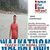## TyroCity is a community of amazing learners

We're a place where students share, stay up-to-date, learn and grow.

# Discussion on: How can a positive NPV end up with negative NPV with the same set of cash flow and time horizonNet present value is the present value of the cash flows at the required rate of return of your project compared to your initial investment,” says Frank Knight (1921). In practical terms, it’s a method of calculating your return on investment, or ROI, for a project or expenditure. By looking at all of the money you expect to make from the investment and translating those returns into today’s Prices, you can decide whether the project is worthwhile

Net present value is based on the concept of equivalent worth of all cash flows to the base point or beginning point in time called the present (Gallo A. , 2016). Net present value (NPV) of a given series of cash flow is the equivalent value/worth at the end of year zero or beginning of year 1. Hence, according to this method all cash inflows and outflows are discounted to the base point at an interest rate for the economic study period in the following manner.

PW(i) = F0(1 + i)0 + F1(1 + i)-1 + F2(1 + i)-2 +…+ FN(1 + i)-N

PW(i) = ∑NK=0 FK (1 + i)-k

Where, i= Effective interest rate or MARR per compounding periods

K= Index for each compounding periods
Fk= Future Cash flow at the end of period K
N = Number of compounding periods in economic study.

Decision Rule

While using the Net Present Value method for economic evaluation of any project, we should consider the following decision rule.

If PW (i) > 0, accept the Project

If PW (i) = 0, remain indifference

If PW (i) < 0, reject the Project

Example (1):

Initial Investment (I) = Rs. 1000000
Annual Revenue ® = Rs. 20000
Annual Expenses (E) = Rs. 5000
Salvage Value (S) = Rs. 25000
Useful Lifecycle = Rs. 5 years
MARR = 10% per year.

Using Net Present value formulation

PW (10%) = -100000 + (20000 - 5000) (P/A, 10%, 10) + 25000 (P/F, 10%, 10)

PW (10%) = -100000 + 15000[(1 + i)N - 1/i(1 + i)N] + 25000 [(1 + i)-N ]

PW (10%) = -100000 + 15000[(1 + 0.1)5 - 1/i(1 + 0.1)5] + 25000 [(1 + 0.1)-5 ]

PW (10%) = Rs. 1807

Since, PW (10%) > 0, the project is acceptable for investment.

Similarly, for other example (2) is

Land cost = Rs. 10, 00000
Building cost = Rs. 1200000
Equipment = Rs. 400000
Sales revenue = Rs. 1500000 per years for 5 years

Salvage values after 5 years will be as follows.
Land = Rs. 2000000
Building = Rs 300000
Equipment = Rs 100000
Annual expenses = Rs. 1000000
MARR = 20%

The Total investment = Rs. (1000000 + 1200000 + 400000) = 2600000
Net annual revenue = Rs. (1500000 - 1000000) = Rs. 500000
Salvage Value = Rs. (2000000 + 300000 + 100000) = Rs. 2400000

Using Net Present value

PW(20%) = - 2600000+ 500000 (P/A, 20%, 5) + 2400000 (P/F, 20%.5)
PW(20%) = - 2600000+ 500000 (4.19247) + 2400000 (0.1615)
PW(20%) = - 2600000 + 2096235 + 387600
PW(20%) = -116165

Since, PW(20%) is negative (i.e. < 0), The proposed plan is rejected to investment.

We can conclude that when we increase the discounted rate of project then the value of Net Present Value is negative. We can clearly visualize from Example (1) and Example (2). More the Discounted rate the PW may be negative and then the proposed plan is rejected. But less the discounted rate the PW may be positive and then the proposed plan is accepted. Higher the rate of IRR the maximum chance to come negative value of present worth in our purposed plan and similarly lower the rate of IRR the maximum chance to come positive value of present value in our purpose plant. So when the NPV comes in positive value then only then purposed plan is accepted. The discount rate will be company-specific as it’s related to how the company gets its funds. It’s the rate of return that the investors expect or the cost of borrowing money. If shareholders expect a 12% return that is the discount rate the company will use to calculate NPV. If the firm pays 4% interest on its debt, then it may use that figure as the discount rate. At this condition the ROI rate is higher than discounted rate so Net Present value is Positive.

There are generally three options available: internal rate of return, payback method, and net present value.Frank Knight (1921) says that net present value, often referred to as NPV, is the tool of choice for most financial analysts. There are two reasons for that. One, NPV considers the time value of money, translating future cash flows into today’s Prices.

References
Frank, K. (1993). Risk, Uncertainty, and Profit. Boston: Houghton Mifflin.

Gallo, A. (2016). A Refresher on Net Present Value. Harvard Business Review.